# Using the Fibonacci numbers to represent whole numbers

First we show how to use the Fibonacci numbers only to represent every whole number, how to use it to convert easily between miles and kilometres, that multiplication in this system is easy and a connection with the Rabbit sequence. All this is done from scratch and uses only school mathematics.
The calculators on this page require JavaScript but you appear to have switched JavaScript off (it is disabled). Please go to the Preferences for this browser and enable it if you want to use the calculators, then Reload this page.
The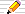icon means there is a You Do The Maths... section of questions to start your own investigations. The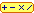calculator icon indicates that there is a live interactive calculator in that section.

## Our decimal system

The way we write our numbers is based on a system of tens - the decimal system. Each column is worth ten times the one on its right so that the columns indicate powers of ten. Here is three thousand, six hundred (no tens) and seven shown in a table with the values of the respective digits shown as the column headings.
 column values column multiplier Sum ... 1000 100 10 1 3 6 0 7 3000 600 7 = 3607

Since each column is TEN times the one on its right, we need ten symbols to represent the ten values in each column: 0, 1, 2, 3, 4, 5, 6, 7, 8 and 9, called digits.

Each positive number has a unique representation in the decimal system. Why use 10? The reason is almost certainly that early writing systems were based on counting using the fingers. [Our word digit comes from the Latin for finger. ] Tally systems were ways of putting marks or notches in wooden sticks (tally sticks) and they can be read more easily if grouped in batches of 5 or 10 for convenience.

## Other bases

What if we used another power or base rather than ten?

### Binary

Using powers of 2, we have the binary system, used in almost all computers. Here the columns are labelled with the powers of 2, and there are just 2 binary digits, 0 and 1, called bits.
 column values column multiplier Sum ... 16 8 4 2 1 1 0 1 1 8 2 1 = eleven
In order to distinguish 11 (eleven) from 11 in another base, we will put the base as a subscript (or sometimes in brackets) after the representation to avoid confusion. So 1011 in binary is 11 in base 10 is written as:
10112 = 1110
Note that the base numbers (here 2 and 10) are always ordinary decimal numbers.
In the next section we will see that the binary system is used in musical notation.

#### Musical Notation

If a crotchet is taken as lasting for one beat, then the semibreve is 4 beats , the minim 2, a quaver 1/2, a semiquaver 1/4 and demi-semiquaver is 1/8. They are written in musical notation as shown here:
NoteSymbolDuration
(beats)
Semi-breve4
Minim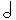2
Crotchet1
Quaver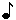1/2
Semiquaver1/4
Demisemiquaver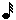1/8
A dot is placed after a note to add on one half of its value. So a dotted crotchet is a crotchet plus a quaver and has a duration of 1·5 time units; two dots after a crotchet give a duration of 1 + 1/2 + 1/4 = 1·75 units.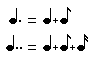• An Introduction to Number Scales and Computers, Longmans, 1965, page 65, says he thinks the record number of dots is 4 in Verdi's Requiem in the Rex Tremenda. It is useful when a long note is followed by a quick note and the next note is "on the beat".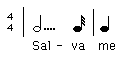Binary fractions are written using column headings as follows:

`   ... 8  4  2  1   ·  1/2  1/4  1/8 ...`
So 1/4 = 0·012 and 3/8 = 0·0112 since it is 1/4+1/8.

In binary, a dot after a crotchet adds a one in a fractional column:

crotchet = 1
crotchet dot = 1·12
crotchet dot dot = 1·112
crotchet dot dot dot = 1·1112
and so on.

### More bases

Base 8 is called octal and is presumably used by intelligent octopuses (or should that be octopi)!
It uses "digits" 0, 1, 2, 3, 4, 5, 6 and 7.
Base 3 is ternary and uses only 0, 1 and 2.
Here is one hundred expressed in all the bases from 2 to 9:
11001002 = 102013 = 12104 = 4005 = 2446 = 2027 = 1448 = 1219 = 10010
Base 2 is called binary,
Base 3 is called ternary,
Base 4 is called quaternary,
Base 5 is called quinary,
Base 6 is called senary,
Base 7 is called septenary,
Base 8 is called octonary or octal,
Base 9 is called nonary,
Base 10 is called denary or decimal,
continued below ...

### Base 1?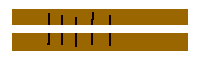Numbers in base 1 have columns that are powers of 1, so they are all ones! But we need column entries of just one kind (since there are B symbols in base B), so we use "1".
You might think that this base is not very good, but it was, in fact, the earliest system of written numbers. In Base 1, 2 is 11, 3 is 111, 4 is 1111, etc. So we make marks (1s) to count the number.
This was used in a Tally System which worked like this: Suppose I lent you 5 sheep to graze on your field and cut your grass, I would make a mark for each sheep on a stick. Then the stick would be broken in half lengthways (across the marks) so we each had a copy of the 5 marks. The two sticks could then be compared to see if they tallied (agreed) to prevent me adding a mark or you erasing a mark on the sticks.
Our English word score for the number of points made by one side in a game also means "to cut". The system was widely used in England from the twelfth century until 1826 by the Exchequer (see Oystein Ore's book below for pictures). When the collection of old wooden tallies was burned in 1836, it caused a fire in the old wooden Parliament buildings in London and burnt them down. The current Houses of Parliament (Westminster Palace) was built to replace them.

### What about bases bigger than 10?

There is no logical reason why we cannot use any integer bigger than zero for a base. The only problem is what to use to represent 10 or more in a single column? We need a single symbol for each value from 0 to B-1 in base B.

Usually the capital letters, A, B, C, etc, are used which take us up to base 36 (using the 10 digits and the 26 letters) - after that, it's up to you!

1010 = A, 1110 = B, 1210 = C, and so on.
Here is one hundred again, this time expressed in some bases bigger than ten:
10010 = 9111 = 8412 = 7913 = 7214 = 6A15
Base 11 is called undenary,
Base 12 is called duodenary or duodecimal,
Base 16 is commonly called hexadecimal - a late 20th century term invented for applications in electronic computers.

Chad Lake at the University of Utah has a nice page on what he calls the Snake Algorithm for converting from one base to another on paper. It is a web page for a course he gave at Indiana University.

###Convert from one Base to another

Base Converter C A L C U L A T O R
 in base 12 (binary)3 (ternary) 4 (quaternary)5 (quinary)6 78 (octal)9 10 (decimal)1112 (duodecimal) 131415 16 (hexadecimal)1718 192021 222324 252627 282930 313233 343536 to base 12 (binary)3 (ternary) 4 (quaternary) 5 (quinary)6 7 8 (octal)910 (decimal) 1112 (duodecimal)13 141516 (hexadecimal) 171819 202122 232425 262728 293031 323334 3536
R E S U L T S: Bases converter Fib sums Zeckendorf reps Think of a number Fib Partitions Neg Index Fib Base Fib2 base

Here is an investigation into representing numbers as sums of Fibonacci numbers. First, let's just use any Fibonacci number once in any of our sums to see what we get.
For example:
• 1 is a sum all on its own! So there is just one sum of Fibonacci numbers with a sum of 1!
• 1+1=2 but we are not allowing this as a Fibonacci sum since we have used 1 more than once!
• 2 is, however, a sum formed of Fibonacci numbers but with just one number all on its own.
• 1+2=3 and 3 is also a Fibonacci number so there are two sums for 3.
• 1+3=4 is the only way to make a total of 4 using only Fibonacci numbers.
• 2+3=5 and again 5 is a Fibonacci number so there are two sums for 5
• Find two sums for 6.
• Find the single sum for 7.
• How many ways can you make 8?
Did you remember that 8 is also a Fibonacci number in the last example above? If so, you will have found three ways to make 8 as a Fibonacci sum.

 1 is the smallest number with just a single number in its Fibonacci sum 3 is the first number with two Fibonacci sums; 8 is the smallest number with three such sums: 1+2+5=3+5=8 ? What is the smallest number with 4 Fibonacci sums? (Hint: It is less than 20) ? What is the smallest number with 5 Fibonacci sums? (Hint: It is less than 30) ... Can you continue this series of smallest numbers with n Fibonacci sums?

You might have noticed that we are assuming ....

### Every number is the sum of some set of Fibonacci Numbers

Note: By set here we mean the mathematical term for a collection of unique items, no item being repeated.
The set {1,3,5} is allowed as a collection of Fibonacci numbers with a sum of 9 because each number in the collection appears no more than once (all the items are unique).
But {2,2,5} is excluded as a set totalling 9 even though all the numbers are Fibonacci numbers because it has a repeated number in it.

This result is indeed true but we do not prove it here. It forms the idea behind representing numbers using Fibonacci numbers that we will investigate now in different forms on the rest of this page.
Our decimal system relies on the fact that Every number is the sum of some collection of Powers Of Ten where we can use each power of ten up to ten times.

You might expect that the more numbers we want in the Fibonacci sum, the larger will be the first number we find with such a sum, and, in general, you would be right. But notice that this series is not always increasing! You will therefore be able to find numbers a and b where a is less than b, but a will have more Fibonacci sums than the larger number b in this list!

###Fibonacci Sums Calculator

Fibonacci Sums C A L C U L A T O R
 of unique Fibonacci numbers whose sum is between and that are the sum of Fibonacci sets
R E S U L T S: Bases converter Fib sums Zeckendorf reps Think of a number Fib Partitions Neg Index Fib Base Fib2 base

####You Do The Maths...

1. What about the number of Fibonacci sums for the Fibonacci numbers themselves?
We have seen the number of Fibonacci sums for 1 and for 2 is 1; for 3 and for 5 is 2; for 8 it is 3. What about 13? and 21? What is the pattern here? Can you explain it?
2. What is special about the number of Fibonacci sums for these numbers:
1,2,4,7,12,20,... ?
What are the next 2 numbers with the same property?
Suggest a simple formula for the numbers in this series. Again, can you prove or explain your result?
3. Find the series of numbers that have just 2 Fibonacci sums. [Hint: it consists of two interleaving series.]
Quite a bit is now known about this series: the number of representations of n as a sum of unique Fibonacci numbers (Sloane's A000119) which is called R(n). Here is a graph of R(n) for n from 1 to 143. At first it looks fairly random, but look closely and you'll find several examples of a fractal pattern where a section of the pattern is repeated but surrounded before and afterwards by another pattern.
Use the buttons on the right to see the graph for n in the ranges 1 to 600 and 1 to 1000.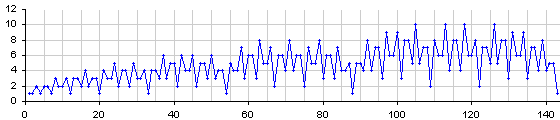If you have a nice explanation or a proof of your answers to the questions above or you find some other patterns, please email me using the address at the bottom of this page.

## The Fibonacci base system

Going back to the decimal number system, what if we labelled the columns with the Fibonacci numbers instead of powers of 10? We follow the usual conventions of larger column sizes being on the LEFT:
... 13 8 5 3 2 1
We will show that a number is represented in this system by putting Fib after it: e.g.:
 8 5 3 2 1 ten = 1 0 0 1 0 Fib = 8 + 2
which distinguishes it from ten thousand and ten (10010) in decimal.

### The Zeckendorf Representation

This time it is not clear what digits we should use in the columns. For instance, there are many ways to represent the value ten in this system as well as in the example above:
 10 = 25 = 2000Fib = 5 + 3 + 2 = 1110Fib = 33 + 1 = 301Fib = 101 = AFib
Usually a number representation system is most useful if it has a unique representation of every integer.

If we use only the digits 0 and 1 then we have our Fibonacci Sums of the previous section. But we saw there that, although each number does have such a sum (i.e. it is representable), some numbers have more than one sum and so their representation is not unique.

We can find a single way to write every number as a sum of Fibonacci numbers if we also have the rule that no two consecutive Fibonacci numbers can be used in the same sum. In terms of the base system with Fibonacci numbers as the headings, this means that no two ones can occur next to each other. This last condition is because the sum of any two consecutive Fibonacci numbers is just the following Fibonacci number, so we can always replace ..011.. by ..100.. .

To convince yourself that every number can be represented in this system, write down the Fibonacci representations of all the numbers from 1 to 40. It starts as follows:

DecimalFibonacci
00
11
210
3100
4101
51000
61001
71010
810000
910001
DecimalFibonacci
1010010
1110100
1210101
13100000
14100001
15100010
16100100
17100101
18101000
19101001
DecimalFibonacci
20101010
21 1000000
22 1000001
23 1000010
24 1000100
25 1000101
26 1001000
27 1001001
28 1001010
29 1010000

### Historical Note on the name "Zeckendorf Representation"

This system is also called the Zeckendorf representation of a number after Edouard Zeckendorf who wrote about it (in French) in 1972. He proved that each representation of a number n as a sum of distinct Fibonacci numbers, but where no two consecutive Fibonacci numbers are used (and there is only one column headed "1"), is unique. He mentions that he proved this in 1939 but he only published it in 1972.
Lekkerkerker had written about this representation (publically) in 1952 in Dutch showing that there is only one way to write a number in this system but, since often it is only when a result is published that it is recognized, the system is now generally called Zeckendorf's.

###Fibonacci Base Calculator: Zeckendorf Representations

C A L C U L A T O R
 up to
R E S U L T S: Bases converter Fib sums Zeckendorf reps Think of a number Fib Partitions Neg Index Fib Base Fib2 base

####You Do The Maths...You Do The Maths...1. The Zeckendorf system uses the set with the fewest Fibonacci numbers in it. What about choosing that set with the most Fibonacci numbers with a sum of n, each Fibonacci number being used at most once? This is called the maximal Fibonacci bit representation. "Bit" means that the only digits in the representations are 0 and 1. Zeckendorf's is therefore the minimal Fibonacci bit representation.
Make a table of the maximal bit representation for n from 1 to 25.
2. 4 has a Zeckendorf representation of 101Fib = 3+1. It is also the only set of Fibonacci numbers with a sum of 4. What other numbers have just a single set of Fibonacci numbers that sum to them?
3. Investigate the number of ones in the Zeckendorf representations. What patterns can you find? Can you express your patterns as mathematical formulae?
4. What about the size of the largest sets (the number of ones in the maximal bit representations)? Is there a formulae for this function (from n to the size of the largest set)?

## Applications of the Fibonacci Number Representation

### Conversion between miles and kilometres

There are approximately 8 kilometres in 5 miles. Since both of these are Fibonacci numbers then there are approximately Phi (1.618..) kilometres in 1 mile and phi (0.618..) miles in 1 kilometres.

The real figure is more like 1.6093.. kilometres in 1 mile. This comes from the precise definition of 1 inch equals 2.54 centimetres exactly, and 100,000 centimetres make 1 kilometre. In the imperial system, 36 inches are 1 yard and 1760 yards are 1 mile.

Replacing each Fibonacci number by the one before it has the effect of reducing it by approximately 0.618 (phi) times (the ratio of a Fibonacci number to the one before it is nearly phi).

So to convert 13 kilometres to miles, replace 13 by the previous Fibonacci number, 8, and 13 kilometres is about 8 miles. Similarly, 5 kilometres is about 3 miles and 2 kilometres is about 1 mile.

Now suppose we want to convert 20 kilometres to miles where 20 is not a Fibonacci number. We can express 20 as a sum of Fibonacci numbers and convert each number separately and then add them up.

Thus 20 = 13 + 5 + 2.
Using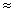to stand for approximately equals and replacing 13 by 8, 5 by 3 and 2 by 1, we have

 20 kms = 13 + 5 + 2 kilometres8 + 3 + 1 miles = 12 miles.
To convert miles to kilometres, we write the number of miles as a sum of Fibonacci numbers and then replace each by the next larger Fibonacci number:

 20 miles = 13 + 5 + 2 miles21 + 8 + 3 kilometres = 32 kilometres.
There is no need to use the Fibonacci Representation of a number, which uses the fewest Fibonacci numbers, but you can use any combination of numbers that add to the number you are converting. For instance, 40 kilometres is 220 and we have just seen that 20 kms is 12 miles. So 40 kms is 212 = 24 miles approximately.

[With thanks to Paul V S Townsend for reminding me of this application.]

####You Do The Maths...You Do The Maths...1. A few years ago, the speed limit in USA was 55 miles per hour (mph). What would that be in kilometres per hour (km/h)?
2. The speed limit on UK motorways is 70 mph. What is this in kilometres per hour?
3. The speed limit in built up areas is 30 mph in the UK. What is 30 mph in km/h?
What do you think the "30" signs would be replaced by if road signs went metric, i.e. round your conversion to the nearest 5 km/h?
4. The current train speed record of 552 km/h was set on April 14 1999 in Japan.
What is the equivalent speed in mph using the Fibonacci method?
What is the equivalent speed in mph using the conversion factor of 1.6093 km per mile?

### A Think Of A Number Magic Trick

This is a magic trick involving cards where the magician asks someone to think of a number and the magician will tell him what it is. The magician hands a set of cards to the person asking them to choose the ones on which his number appears. Since there are many numbers on each card it is not easy to see which are common to all the cards but nevertheless, the magician immediately announces the number thought of.

To show what is happening, here are a set of 4 cards where the number to be thought of is between 1 and 12:
Fibonacci Base
n85321
1....1
2...10
3..100
4..101
5.1000
6.1001
7.1010
810000
910001
1010010
1110100
1210101
 A1 4 6 9 12 B2 7 10 C3 4 11 12 D5 6 7 E 8 9 10 11 12
As the magician, the secret of the trick is to add up the first (smallest) number on each card that is handed back to you and that is the number thought of.
So suppose I thought of 9. I would hand back to you cards A which start with 1 and card E which starts with 8.
You would add them and announce that 9 was the number I thought of.

The trick works by listing on the first card all those number with a 1 in the units column of its Fibonacci base representation.
The second card contains all those numbers with a 1 in the second column; the third card all those with a 1 in the third column; and so on.

The trick looks much more impressive if there are many numbers on each card as it fairly easy in the small example above to spot the common number on the cards handed to you.
So here is a calculator to produce a set of cards for any range of numbers you ask someone to chose from:

###Think of a Number Card Generator

C A L C U L A T O R
 Think of a number between 1 and

R E S U L T S: Bases converter Fib sums Zeckendorf reps Think of a number Fib Partitions Neg Index Fib Base Fib2 base

## An easy way to Multiply

### The Egyptian system - using Doubling...

The Egyptians had an easy way to multiply two integers which involved only doubling numbers and adding - no multiplication tables to learn and no need for a calculator (except to do the addition).
For example, 19 x 65. We write the two numbers at the head of two columns, choosing one column to keep doubling and the other to keep halving ignoring remainders, until the halving column reaches 1:
```  halve double odd?
19    65    +
9   130    +
4   260
2   520
1  1040    +
```
Any row whose halving column entry was odd is marked (here with +) and we add the corresponding values from the doubling column.
In our example 65+130+1040=1235 which is the product of 19 and 65.
The method works because if we represent 19 in the binary system we have 16+2+1=10011(2) and so 19x65=(16+2+1)x65 which is 16*65 + 2*65 + 1*65. ie, the 1st, 2nd and 5th values in the doubling column above.

####You Do The Maths...

1. Check that if you halve the 65 column and double the 19 column the method still works.
2. Try the Egyptian method on 32x65.
3. Try it on 31x65.

### The Fibonacci system

A similar system uses the Fibonacci representation to replace each doubling of the Egyptian method with an addition.

Let's take the same example: 19x65.
This time we take just one number - say 65 - as the head of the right hand column, the left column starting with 1. The second row has 2 on the left and we double 65 to get 130 on the right. Now each successive row is the sum of the previous TWO entries above it, taking each column separately. So since we started with 1 and 2 on the left we will get 3,5,8,... that is, the Fibonacci numbers on the left hand side. Stop when we can find a Fibonacci number which is bigger than the other number in the product - here 19:

```   1   65 +
2  130
3  195
5  325 +
8  520
13  845 +
21
```
We mark the rows this time by finding those entries in the left column that add up to 19. There many be several ways to do this selection but any will do. Here we have chosen 13+5+1. If we add up the right hand entries on these rows we have: 65+325+845=1235 which is again 19x65.

####You Do The Maths...

1. Try it the other way round, starting with 19 and stop when the Fibonacci number exceeds 65.
2. Try the same multiplications as above: 32x65 and 31x65.
3. Look up the article where this idea was first presented:
Fibonacci, Lucas and the Egyptians by S La Barbera in The Fibonacci Quarterly, Vol 9, 1971, pages 177-187.

## Patterns in the Fibonacci representations

### Patterns in the columns - the Rabbit sequence

In base 10, if we list all the integers from 1, then there are patterns in the columns:

#### Decimal patterns

Column 1 (units) cycles through all the digits 0, 1, 2, 3, 4, 5, 6, 7, 8 and 9 repeatedly;
Column 2 (tens) cycles through all the digits but each digit occurs ten times;
Column 3 (hundreds) is the same but each digits occurs 100 times;
and so on.

#### Fibonacci Representations patterns

Is there a pattern in the columns of numbers in the Fibonacci base system?
Yes there is!
It is based on the Rabbit sequence which now includes the initial 0.
The pattern in column one (the right-hand column) is derived from the rabbit sequence where
every "1" in the rabbit sequence has been replaced by "10":-
The rabbit sequence:
`010110101101101011010... `
becomes:
```  0 1  0 1  1  0 1  0 1  1  0 1  1  0 1  0 1  1  0 1  0 ...
0 10 0 10 10 0 10 0 10 10 0 10 10 0 10 0 10 10 0 10 0 ... ```
which is column 1 above, read downwards.

[N.B. This is exactly the same as if we flipped the bits (1 changes to 0 and 0 to 1) in the Rabbit sequence (without its initial zero)!! However, there is a pattern in the other columns which is better seen with the description above.]

What about column 2 of the Fibonacci representations?
This is derived similarly:
every "1" in the rabbit sequence is replaced by "100" and
every "0" is replaced by "00".
```  0  1   0  1   1   0  1   0  1   1   0  1   1   0  ... Rabbit Sequence
00 100 00 100 100 00 100 00 100 100 00 100 100 00 ... Column 2```
where column 2 in the Table of Fibonacci representations is read downwards.

For column 3, replace "0" by "000" and "1" by "11000"
For column 4, replace "0" by "00000" and "1" by "11100000"
For column 5, replace "0" by "00000000" and "1" by "1111100000000"

The same pattern follows for all the columns:

Column i the just the rabbit sequence with
"0" replaced by F(i) 0s and
"1" replaced by F(i-1) 1s followed by F(i) 0s.
DecimalFibonacci
00
11
210
3100
4101
51000
61001
71010
810000
910001
1010010
1110100
1210101
13100000
14100001
15100010
16100100
17100101
18101000
19101001
20101010

### The number of 1s in a Fibonacci Representation

What is the least number of Fibonacci numbers that sum to a given n?
This is the number of 1s in the Fibonacci representation, since the description given above guarantees the least number of Fibonacci's and is also called the minimal Fibonacci representation. Here we repeat the Fibonacci Representation table from above but now include the number of 1's in each representation:
nnFib1's
1 11
2 101
3 1001
4 1012
5 10001
6 10012
7 10102
8 100001
9 100012
10 100102
11 101002
12 101013
From the table, we can see that the number of numbers with a Fibonacci representation of a given length is a Fibonacci number:
There is 1 of length 1,
there is 1 of length 2,
there are 2 of length 3,
there are 3 of length 4,
there are 5 of length 5,...

Here is a more compact list of the number of 1s in the (minimal) Fibonacci representation of the first few whole numbers :
 1 2 3 4 5 6 7 8 9 10 11 12 13 14 15 16 17 18 19 20 21 22 23 24 25 26 27 28 29 30 31 32 33 ... 1 1 1 2 1 2 2 1 2 2 2 3 1 2 2 2 3 2 3 3 1 2 2 2 3 2 3 3 2 3 3 3 4 ...
If we split this list into sublists corresponding to the different lengths of Fibonacci representations we have the following:

 1=1Fib 1, 2=10Fib 1, 3=100Fib,4=101Fib 1,2 5=1000Fib, 6=1001Fib, 7=1010Fib 1,2,2 8, 9, 10, 11 and 12 1,2,2,2,3 13 to 20 1,2,2,2,3,2,3,3 21 to 33 1,2,2,2,3,2,3,3,2,3,3,3,4 34 to 54 1,2,2,2,3,2,3,3,2,3,3,3,4,2,3,3,3,4,3,4,4 ... ...
It is quite easy to see where this pattern comes from: Each time we put a 1 at the start of our Fibonacci representations and then copy the earlier patterns. For example, 8, 9, 10, 11 and 12 are 8+0, 8+1, 8+2, 8+3 and 8+4.

Can you see any patterns in these sequences?
It seems that each sequence starts off the following sequence.
Can you discover how the remainder of each is formed, that is, the part that follows (the copy of) the previous sequence? It is not quite the sequence before, but, one added to all the items of the sequence before:

The next sequence is the preceding one followed by adding one to the sequence before the preceding one.
Since each sequence in the list above starts off the following one, it defines a unique infinite sequence.

### Palindromic Fibonacci Representations

A palindrome is a word or list that is the same when reversed, e.g. radar or 1001.
Here is the start of the list of numbers which are palindromes in the Fibonacci representation:
N Fib rep of N
11
4101
61001
910001
1210101
14100001
221000001
271001001
331010101
3510000001
5110100101
56100000001
64100010001
74100101001
80101000101
88101010101
901000000001
Is there a formula for the series 1,4,6,9,12,14,... A094202?

## A more general Fibonacci System using Partitions

Suppose that, instead of finding just a set of Fibonacci numbers that sum to N, that is each Fibonacci number is included at most once in the representation, we allow multiples of any Fibonacci number. We will then have a multi-set or bag of Fibonacci numbers whose sum is N, also called a partition of n.
It is the same as using the Fibonacci Base system above but removing the restriction that entries in the columns must be only 0 and 1. We could call the system above the Binary Fibonacci Representation to distinguish it and, if there are no two consecutive 1s in the representation then it is a Zeckendorf Representation.
We can now use 0 and any positive whole number in any column. The table below illustrates this for the values 1 to 6. Notice that each of these representations is just a partition on n using only the Fibonacci numbers.
A partition is a sum of whole numbers with a given total where the order of the components in the partition does not matter:
for example 1+1+2 is the same partition of 4 as 1+2+1

NFib numbers whose sum is N
allowing repeats
Count
111
22, 1+12
33, 2+1, 1+1+13
43+1, 2+2, 2+1+1, 1+1+1+14
55, 3+2, 3+1+1, 2+2+1, 2+1+1+1, 1+1+1+1+16
65+1, 3+3, 3+2+1, 3+1+1+1, 2+2+2, 2+2+1+1, 2+1+1+1+1, 1+1+1+1+1+18
1. Since we can have just a single use of a Fibonacci number (no repeats) in this new system, all the Zeckendorf representations that we looked at above are also included in the table. Every number has a Zeckendorf representation which is given first.
2. There are more sets of Fibonacci numbers that sum to N (i.e. where no Fibonacci number is used more than once) than the Zeckendorf representation. In the Zeckendorf representation no two consecutive Fibonacci numbers are used. We can have any Fibonacci numbers in our bags and so this time we do allow neighbouring Fibonacci numbers in any collection (bag).
3. We are only interested in the collection of Fibonacci numbers that sum to n, where we allow repeated use of any Fibonacci number. For example 5 is 2+2+1 and this is the same collection or bag as 1+2+2 and 2+1+2, since each includes two 2's and one 1.

###A Fibonacci Partitions Calculator

C A L C U L A T O R
 of up to
R E S U L T S: Bases converter Fib sums Zeckendorf reps Think of a number Fib Partitions Neg Index Fib Base Fib2 base

### The Fibonacci Partition Representation

Using bags and partitions of Fibonacci numbers that sum to n, and the existence of at least one bag for every number n, means we now have another system of representing numbers: the Fibonacci Partition Representation.
Collecting the frequencies of each Fibonacci number in a particular bag or partition gives a Fibonacci Partition representation of that bag or sum.
The columns in this system are again the Fibonacci numbers from 1 upwards (1 only occurring once). The entries in the columns are the frequencies of that Fibonacci number in a bag with a sum of n. This 13 is the sum of the Fibonacci bag 5,2,2,2,1,1 and so is written as
 ... 5 3 2 1 1 0 3 2 Fib = 13
From the table above we see that 13 has a total of 41 Fibonacci Partition representations.
Here are all the partitions of 6 using Fibonacci numbers and the corresponding Fibonacci Partition representation of 6:
Fibonacci Partition representations of 5
PartitionFib Partition Rep
5321
1+1+1+1+1 5
2+1+1+1 1 3
2+2+1 2 1
3+1+1 1 0 2
3+2 1 1 0
5 1 0 0 0

### How many Fibonacci Partition representations of N are there?

Any number n is just the sum of n 1's and F(2)=1 so that's one bag of Fibonacci's that sum to n, no matter what n is.
All the sets of Fibonacci numbers that sum to n are also included in the bags, since a set is a special kind of bag with no items repeated in it.

Here is a count of the number of Fibonacci Partition representations with a sum from 1 to 40:

 N No. ofreps N No. ofreps N No. ofreps N No. ofreps 1 2 3 4 5 6 7 8 9 10 1 2 3 4 6 8 10 14 17 2211 12 13 14 15 16 17 18 19 20 27 33 41 49 59 71 83 99 115 13421 22 23 24 25 26 27 28 29 30 157 180 208 239 272 312 353 400 453 50931 32 33 34 35 36 37 38 39 40 573 642 717 803 892 993 1102 1219 1350 1489
The series 1, 2, 3, 4, 6, 8, 10, 14, 17,... is A003107 in Neil Sloane's Online Encyclopedia of Integer Sequences.
Advanced note: The generating function for this series is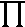i = 2 1 = 1 + x + 2x2 + 3x3 + 4x4 + 6x5 + ...1 – xFib(i)

## Using only the Negatively Indexed Fibonacci numbers

The only restriction with the Fibonacci representations above - the Fibonacci Binary and Zeckendorf as well as the Fibonacci Partition systems - is that they only represent positive numbers. But the Fibonacci series extends "backwards" too to include negative numbers:
 i Fib(i) ... -5 -4 -3 -2 -1 0 1 2 3 4 5 ... ... 5 -3 2 -1 1 0 1 1 2 3 5 ...
Surprisingly, for any number n, we can find a set of Fibonacci numbers all with negative Fibonacci indices, whose sum is n and this applies to both positive and negative n.
We don't need a "0" column so the indices we use will be from –1 downwards for the Fibonacci numbers 1, –1, 2, –3, 5, –8, ... . We can conveniently write the numbers with indices increasing to the right (getting more negative to the left) as in other more conventional number bases systems.
Since we are using a set of negatively indexed Fibonacci numbers, the column entries (the 'digits') will be only 0s and 1s, so we have a binary system again.

For instance 5 = Fib(-5) so we can represent it as

 i Fib(i) 5= -6 -5 -4 -3 -2 -1 -8 5 -3 2 -1 1 0 1 0 0 0 0
There are many other equally valid all-negative-indexed Fibonacci representations too. For instance: Notice that since Fib(n)=Fib(n–1) + Fib(n–2), then "001 " in this representation can always be replaced by "110" and vice-versa. But since all representations begin with "1", which is the same as "001", then we can always expand the left-most 1 into "110" and then expand the left most one again.This leads to infintely many representations for any number.
A convenient way to stop this, and to give a unique representation for every n both positive and negative, is to prohibit "11" in any negatively indexed Fibonacci representation.
When Bunder first described this system (see reference below), he pointed out that we can find such a representation with no consecutive 1s, so it is a variation of the Zeckendorf representation.

Now we have the following examples of Negatively Indexed Fibonacci Representations and we will write (-Fib) after such a representation to distinguish it from the others on this page (and from true binary of course also).

 i Fib(i) -7 -6 -5 -4 -3 -2 -1 13 -8 5 -3 2 -1 1 -1 . . . . 1 0 -2 . . 1 0 0 1 -3 . . 1 0 0 0 -4 . . 1 0 1 0 -5 1 0 0 1 0 1 -6 1 0 0 1 0 0 -7 1 0 0 0 0 1 -8 1 0 0 0 0 0 -9 1 0 0 0 1 0 -10 1 0 1 0 0 1 -11 1 0 1 0 0 0 -12 1 0 1 0 1 0

 i Fib(i) -7 -6 -5 -4 -3 -2 -1 13 -8 5 -3 2 -1 1 1 . . . . . . 1 2 . . . . 1 0 0 3 . . . . 1 0 1 4 . . 1 0 0 1 0 5 . . 1 0 0 0 0 6 . . 1 0 0 0 1 7 . . 1 0 1 0 0 8 . . 1 0 1 0 1 9 1 0 0 1 0 1 0 10 1 0 0 1 0 0 0 11 1 0 0 1 0 0 1 12 1 0 0 0 0 1 0

###Negatively Indexed Fibonacci Base Convertor

C A L C U L A T O R
 up to -Fib to base 10

R E S U L T S: Bases converter Fib sums Zeckendorf reps Think of a number Fib Partitions Neg Index Fib Base Fib2 base

## The Fibonacci2 Base system

We use the squares of the Fibonacci numbers as the columns for a base Fib2 representation of integers too.
 Index i: Fib(i): Fib(i)2: 1 2 3 4 5 6 7 8 1 1 2 3 5 8 13 21 1 1 4 9 25 64 169 441
In other words, is there a collection of the squares of Fibonacci numbers that sums to n for each whole number n?
There are two 1s already in the list of squares of Fibonacci numbers and we can use both of them for a collection with a total of 2, but there is no collection of these squares that has a sum of 3.
However, if we use each square Fibonacci number at most twice and use the two 1's in the Fibonacci sequence, then we can find ways to represent every whole number n.
As usual, we list the columns in increasing order from right to left:
i: Fib(i): Fib(i)2: 1 ... 3 2 1 ... 2 1 1 ... 4 1 1 1 1 0 2 1 1 2 0 2 1 1 2
 i: Fib(i): Fib(i)2: 7 12 127 ... 6 5 4 3 2 1 ... 8 5 3 2 1 1 ... 64 25 9 4 1 1 1 2 1 1 1 2 1 0 2 1 1 0 1 2 2 2 2 1 2 1 1 0 0 1 2 1 0 2 2

### The Balanced Fibonacci2 Representation

Instead of using the digits 0, 1 and 2, we could use -1, 0 and 1, called the balanced representation.
All numbers have a balanced representation
Because they all have a 0,1,2 representation and we can use these to find all the 1,0,T representations for a given number n.
We take any 0,1,2 Fib2 representation and subtract from it the number "11...111" with the same length.
This transforms 0→-1, 1→0 and 2→1.
We are only interested in 10T representations that begin with 1 (or T is the value is negative).
We can thus find
• the 0,1,2 representations of n+2 of length 2 beginning "2" and take from each 11Fib2 = 210;
• the 0,1,2 representations of n+6 of length 3 beginning "2" and take from each 111Fib2 = 610;
• any 0,1,2 representations of n+15 of length 4 beginning "2" and take from each 1111Fib2 = 1510;
• any 0,1,2 representations of n+40 of length 5 beginning "2" and take from each 11111Fib2 = 4010;
• and so on
Eventually the representations will become too long to have any representation of the stated length and beginning "2" and we can stop.

For example:
3+2 is 5 which is 110 or 101 in base Fib2 and there are none here of length 2;
3+6 is 9 which is 1000, 210 or 201 in base Fib2 and there are two here of length 3 that begin with 2;
these give the 10T representations 210-111=10T and 201-111=1T0;
3+15 = 18 which in base Fib2 is 2000, 1210 and 1201, all of length 4 but only 2000 begins with 2.
Subtracting 1111 from 2000 gives 1TTT.
3+40 has no representations of length 5 beginning "2".
Therefore the representations of 3 in base balanced Fib2 are 10T, 1T0 and 1TTT.

and we have the added advantage that we can now represent negative numbers too in this notation.
Since we retain the two "1" columns for F(1)2 = 1 = F(2)2. Also we keep to using one symbol (digit) per column so we use the "bar 1" notation for -1, that is, we put the minus sign above the 1 to make 1 or, more conveniently, we use the letter "T".

So, for example, here are some numbers and their representations in the balanced Fibonacci-squared system:

 9 4 1 1 value 1 T 0 1 9 -4 +1 = 5 1 T 1 0 9 -4 +1 = 5 T 1 1 1 -9 +4 +1 +1 = -3

Here is a comparison of the two representations

nFib2 repsbal Fib2 reps
000, 1T, T1
110, 110, 1
220, 11, 21TT, 11
321, 121TTT, 10T, 1T0
4100, 221T0T, 1TT0, 11T, 100, 1T1
5110, 1011T1T, 1T00, 1TT1, 110, 101
6120, 111, 1021T10, 1T01, 111
7121, 11210TT, 1T11
8200, 122100T, 10T0
91000, 210, 201101T, 1000, 10T1
101010, 1001, 220, 211, 2021TTTT, 1010, 1001
111020, 1011, 1002, 221, 2121TT0T, 1TTT0, 11TT, 1011
121021, 1012, 2221TT1T, 1TT00, 1TTT1, 110T, 11T0

###Base Fibonacci2 Calculator

The Fibonacci2 Base system C A L C U L A T O R
 in a column as a list the Fib2 representations of up to
with digits 0,1,2:with digits 0,1,T:
 from base Fib2 to base 10
R E S U L T S: Bases converter Fib sums Zeckendorf reps Think of a number Fib Partitions Neg Index Fib Base Fib2 base

### The Number of Representations Fractal

#### With 0,1,2 digits

There are
2 ways to represent 1: 1 and 10 (Fib2);
3 ways to represent 2: 2, 11 and 20(Fib2);
2 ways to represent 3: 21 and 12.
The number of representations for 1,2,3,4,5,6,7,8 is 2,3,2,2,2,3,2,2 and the complete sequence of counts is A147561.

The number of representations has some interesting fractal properties as shown in these graphs:

 1-  65 1- 250 1- 550 1-1500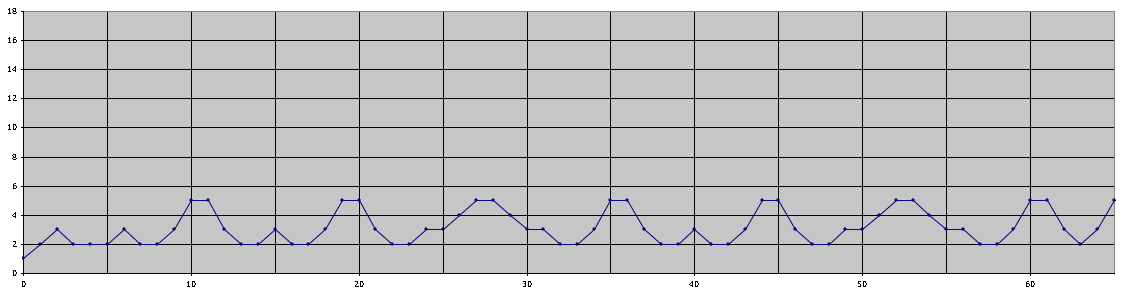Show Graphs: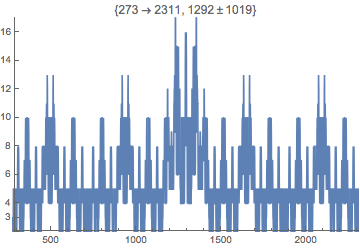allsame height 2: 10-11: 72: 188-9: 493-4: 1292:
with
fixed
scale
2:
10-11:
72:
188-9:
493-4:
1292:

The Calculator in the previous section will give the actual counts shown in the graphs above.

#### With 1,0 T digits

There are
2 ways to represent 1: 1 and 10 (balanced Fib2);
2 ways to represent 2: 1TT, 11 (balanced Fib2);
3 ways to represent 3: 1TTT, 10T, 1T0.
The number of representations for 1 up t0 20 is 2,2,3,5,5,3,2,2,3,3,4,5,5,4,3,3,2 and the complete sequence of counts is ??.

The number of balanced> Fib2 representations also has some interesting fractal properties as shown in these graphs:

 1-  120 1- 350 1- 1700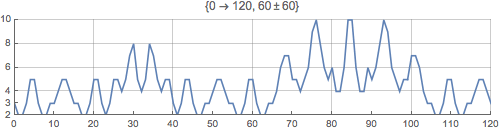Show Graphs:with
fixed
scale
0-9
2-23:
0-64:
2-167:
0-441:
2-1154:

The Calculator in the previous section will give the actual counts shown in the graphs above.

### Brown's Criterion for a Number Representation System

In general, any series of numbers that starts with 1 and that has the property that every number in it does not exceed 1 plus the sum of all the earlier numbers also has the property of being a complete series, meaning its values can be used as columns in a binary number base system (using digits 0 and 1) to represent every whole number.
This is called Brown's Criterion.
But our Fibonacci2 system has digits 0,1 and 2!
So if we take two copies of the set of Fibonacci numbers squared, we will find that Brown's Criterion does apply, as Honsberger shows in the reference below. This means the column headers are the squares of the Fibonacci numbers 1,1,2,3,... and the digits in the columns are 0, 1 or 2.

### Base Fibonaccik Systems

V Hoggatt Jr and Bob CHow proved that we can form a complete base system using the k-powers of the Fibonacci numbers if we have not only 2 copies of the squares, as above, but also with 4 copies of the cubes, 8 copies of the fourth powers, 16 of the fifth powers and so on, that is with 2k–1 copies of the k-th powers of the Fibonacci numbers {1,1,2,3,5,8,...}

## Some more representations using Fibonacci Numbers

Here are some more research topics and investigations on other types of collections of Fibonacci numbers.

###You Do The Maths...

1. We can also look at other kinds of Fibonacci representations. For both sets and bags, it is the items in the collection (and the number of them) that matters, the order in which they are listed begin immaterial since 1,2,3 is the same set (and bag) as 3,1,2.
If the order of elements in the collection now does matter we would be studying sequences of Fibonacci numbers whose sum is N also called compositions. List the number of compositions of n that contain only Fibonacci numbers.
2. Alternatively, we could look at sets but without the Zeckendorf restriction. Such sets (of unique items) could now contain consecutive Fibonacci numbers.
On a later page we will investigate what happens if instead of using the Fibonacci numbers as column headers we use powers of Phi (1.61803..), i.e. base Phi.

## References

• Number Theory and Its History O Ore, Dover (1988), 388 pages, is an excellent and classic book to get you into all aspects of the mathematics of whole numbers (or Number Theory).
On the number of Fibonacci Representations:
• Representations of N as a sum of distinct elements from special sequences D. A. Klarner, Fibonacci Quarterly, vol 4 (1966), pages 289-306 and 322.
• Fibonacci Representations L Carlitz Fibonacci Quarterly vol 6 (1968), pages 163-220 is a long and interesting article about R(n), some recursive formulae and many formulae for special cases of R(n).
• The smallest positive integer having Fk representations as sums of distinct Fibonacci numbers Marjorie Bicknell-Johnson in Applications of Fibonacci Numbers Vol 8 (Proceedings of the Eighth International Research Conference of Fibonacci Numbers and their Applications, Rochester, USA, June 1998, editor F T Howard, Kluwer Academic, pages 47-52 solves the problem of a formula for R(n), the number of sets of Fibonacci numbers whose total is n, by giving a recursive definition for it. The article is followed by two others, the second of which is...
• Composing with Sequences:... but is it art? by John Bliss, in Applications of Fibonacci Numbers Vol 8 (Proceedings of the Eighth International Research Conference of Fibonacci Numbers and their Applications, Rochester, USA, June 1998, editor F T Howard, Kluwer Academic), pages 61-73, is about turning R(n) directly into music.
On Zeckendorf Representations
• Voorstelling van natuurlijke getallen door een som van getallen van Fibonacci C. G. Lekkerkerker, Simon Stevin vol. 29 (1952) pages 190 - 195
• Représentation des nombres naturels par une somme de nombres de Fibonacci ou de nombres de Lucas, E Zeckendorf, Bulletin de las Societe Royale des Science de Liege vol 41 (1972) pages 179-182.
• A Generalized Fibonacci Numeration E Zeckendorf Fibonacci Quarterly vol 10 (1972) page 365-372 with Errata Fibonacci Quarterly vol 11 (1973) page 524.
• Edouard Zeckendorf C Kimberling Fibonacci Quarterly 36 (1998), pages 416-418.
Application of Fibonacci Representation
• Concrete Mathematics (2nd edition) by Graham, Knuth and Patashnik, Addison-Wesley, section 6.6.
On the Fibonacci "Think of a Number" trick
• Fibonacci Magic Cards Brother Alfred Brousseau, Fibonacci Quarterly 10 (1972), pages 197-198
The Negatively Indexed Fibonacci representation was first described in:
• Zeckendorf Representations using Negative Fibonacci Numbers M A Bunder Fibonacci Quarterly 30 (1992) pages 111-115.
Fibonacci2 Representation
Brown's Criterion
• Note on Complete Sequences of Integers, J L Brown Jr, Amer Math Monthly, vol 68 (1961), pages 557-560
On Representations of Numbers using Fibonacci Numbers
• Some Theorems on Completeness V E Hoggatt Jr, B Chow, Fib Quart 10 (1972) pages 551-554, 560.
• Mathematical Gems III Ross Honsberger, Math. Assoc. of Amer. in section 15 of Chapter 8 "A Second Look at the Fibonacci and Lucas Numbers".
A very useful free book
• A Primer for the Fibonacci Numbers: Part XII, V E Hoggatt Jr, N Cox, M Bicknell in Fibonacci Quarterly, vol 11 (1973), pages 317-331 (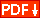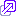)
is a useful introduction to results in this area, but is at mathematics undergraduate level. He uses a binary notation and therefore duplicates each Fibonacci square column whereas here we just have two columns labelled "1" since Fib(1)2 = Fib(2)2=1. He also has lots of results about the symmetry and fractal properties of graph of the number of representations of n but remember that his representations are different, using only 1s and 0s and each column duplicated so there is considerable redundancy in that method.© 1996-2016 Dr Ron Knott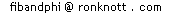updated 29 September 2015